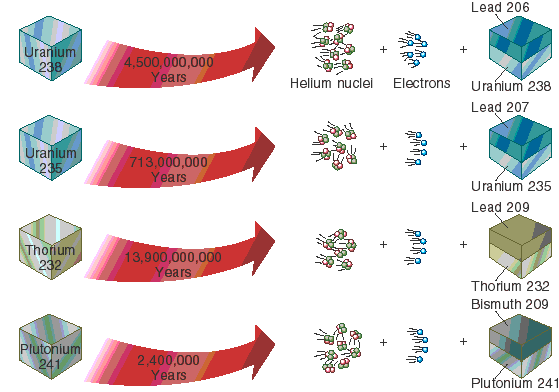# What Is Radioactive Dating, and How Does It Work?

Radiometric dating (often called radioactive dating) is a technique used to date materials such as rocks or carbon, usually based on a comparison between the observed abundance of a naturally occurring radioactive isotope and its decay products, using known decay rates.

The use of radiometric dating was first published in 1907 by Bertram Boltwood and is now the principal source of information about the absolute age of rocks and other geological features, including the age of the Earth itself, and can be used to date a wide range of natural and man-made materials.

### Read about How do we know the Age of the Earth?

As radioactive Parent atoms decay to stable daughter atoms (as uranium decays to lead) each disintegration results in one more atom of the daughter than was initially present and one less atom of the parent.What Is Radioactive Dating, and How Does It Work?

The probability of a parent atom decaying in a fixed period of time is always the same for all atoms of that type regardless of temperature, pressure, or chemical conditions. This probability of decay is the decay constant. The time required for one-half of any original number of parent atoms to decay is the half-life, which is related to the decay constant by a simple mathematical formula.

All rocks and minerals contain long-lived radioactive elements that were incorporated into Earth when the Solar System formed. These radioactive elements constitute independent clocks that allow geologists to determine the age of the rocks in which they occur. The radioactive parent elements used to date rocks and minerals are:

Radiometric dating using the naturally-occurring radioactive elements is simple in concept even though technically complex. If we know the number of radioactive parent atoms present when a rock formed and the number present now, we can calculate the age of the rock using the decay constant.

The number of parent atoms originally present is simply the number present now plus the number of daughter atoms formed by the decay, both of which are quantities that can be measured. Samples for dating are selected carefully to avoid those that are altered, contaminated, or disturbed by later heating or chemical events.

In addition to the ages of Earth, Moon, and meteorites, radiometric dating has been used to determine ages of fossils, including early man, timing of glaciations, ages of mineral deposits, recurrence rates of earthquakes and volcanic eruptions, the history of reversals of Earth's magnetic field, and the age and duration of a wide variety of other geological events and processes.

#### The age equation

The mathematical expression that relates radioactive decay to geologic time is
D = D0 + N(t) (eλt − 1)
where
t is age of the sample,
D is number of atoms of the daughter isotope in the sample,
D0 is number of atoms of the daughter isotope in the original composition,
N is number of atoms of the parent isotope in the sample at time t (the present), given by N(t) = Noe-λt, and
λ is the decay constant of the parent isotope, equal to the inverse of the radioactive half-life of the parent isotope times the natural logarithm of 2.
The equation is most conveniently expressed in terms of the measured quantity N(t) rather than the constant initial value No.
The above equation makes use of information on the composition of parent and daughter isotopes at the time the material being tested cooled below its closure temperature. This is well-established for most isotopic systems.  However, construction of an isochron does not require information on the original compositions, using merely the present ratios of the parent and daughter isotopes to a standard isotope. Plotting an isochron is used to solve the age equation graphically and calculate the age of the sample and the original composition.